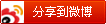# Javassist 使用指南（三）

## 5. 字节码操作

Javassist 还提供了用于直接编辑类文件的低级级 API。 使用此 API之前，你需要详细了解Java 字节码和类文件格式，因为它允许你对类文件进行任意修改。

### 获取 ClassFile 对象

javassist.bytecode.ClassFile 对象表示类文件。要获得这个对象，应该调用 CtClass 中的 getClassFile() 方法。

``````BufferedInputStream fin
= new BufferedInputStream(new FileInputStream("Point.class"));
ClassFile cf = new ClassFile(new DataInputStream(fin));
``````

ClassFile 对象可以写回类文件。ClassFile 的 write() 将类文件的内容写入给定的 DataOutputStream。

### 5.2 添加和删除成员

ClassFile 提供了 addField()，addMethod() 和 addAttribute()，来向类添加字段、方法和类文件属性。

### 5.3 遍历方法体

``````ClassFile cf = ... ;
MethodInfo minfo = cf.getMethod("move");    // we assume move is not overloaded.
CodeAttribute ca = minfo.getCodeAttribute();
CodeIterator ci = ca.iterator();
``````

CodeIterator 对象允许你逐个访问每个字节码指令。下面展示了一部分 CodeIterator 中声明的方法：

• void begin（）
移动到第一条指令。
• void move（int index）
移动到指定位置的指令。
• boolean hasNext（）
是否有下一条指定
• int next（）
返回下一条指令的索引。注意，它不返回下一条指令的操作码。
• int byteAt（int index）
返回索引处的无符号8位整数。
• int u16bitAt（int index）
返回索引处的无符号16位整数。
• int write（byte [] code，int index）
在索引处写入字节数组。
• void insert（int index，byte [] code）
在索引处插入字节数组。自动调整分支偏移量。

``````CodeIterator ci = ... ;
while (ci.hasNext()) {
int index = ci.next();
int op = ci.byteAt(index);
System.out.println(Mnemonic.OPCODE[op]);
}
``````

### 5.4 生成字节码序列

`Bytecode`对象表示字节码指令序列。它是一个可扩展的字节码数组。

``````ConstPool cp = ...;    // constant pool table
Bytecode b = new Bytecode(cp, 1, 0);
b.addIconst(3);
b.addReturn(CtClass.intType);
CodeAttribute ca = b.toCodeAttribute();
``````

``````iconst_3
ireturn
``````

Bytecode 提供了许多方法来添加特定的指令，例如使用 addOpcode() 添加一个 8 位操作码，使用 addIndex() 用于添加一个索引。每个操作码的值定义在 Opcode 接口中。
addOpcode() 和添加特定指令的方法，将自动维持最大堆栈深度，除非控制流没有分支。可以通过调用 Bytecode 的 getMaxStack() 方法来获得这个深度。它也反映在从 Bytecode对象构造的 CodeAttribute 对象上。要重新计算方法体的最大堆栈深度，可以调用 CodeAttribute 的 computeMaxStack() 方法。

### 5.5 注释（元标签）

Javassist还允许您通过更高级别的API访问注释。 如果要通过CtClass访问注释，请在CtClass或CtBehavior中调用getAnnotations（）。

## 6. 泛型

Javassist 的低级别 API 完全支持 Java 5 引入的泛型。但是，高级别的API（如CtClass）不直接支持泛型。

Java 的泛型是通过擦除技术实现。 编译后，所有类型参数都将被删除。 例如，假设您的源代码声明一个参数化类型 Vector<String>：

``````Vector<String> v = new Vector<String>();
:
String s = v.get(0);
``````

``````Vector v = new Vector();
:
String s = (String)v.get(0);
``````

``````public class Wrapper<T> {
T value;
public Wrapper(T t) { value = t; }
}
``````

``````public interface Getter<T> {
T get();
}
``````

``````public Object get() { return value; }
``````

``````Wrapper w = ...
String s = (String)w.get();
``````

## 7.可变参数

``````public int length(int... args) { return args.length; }
``````

``````CtClass cc = /* target class */;
CtMethod m = CtMethod.make("public int length(int[] args) { return args.length; }", cc);
m.setModifiers(m.getModifiers() | Modifier.VARARGS);
cc.addMethod(m);
``````

``````length(new int[] { 1, 2, 3 });
``````

``````length(1, 2, 3);
``````

## 8. J2ME

``````m.getMethodInfo().rebuildStackMapForME(cpool);
``````

## 9.装箱/拆箱

Java 中的装箱和拆箱是语法糖。没有用于装箱或拆箱的字节码。所以 Javassist 的编译器不支持它们。 例如，以下语句在 Java 中有效：

``````Integer i = 3;
``````

``````Integer i = new Integer(3);
``````

## 10. 调试

``````CtClass.debugDump =“./dump”;
``````